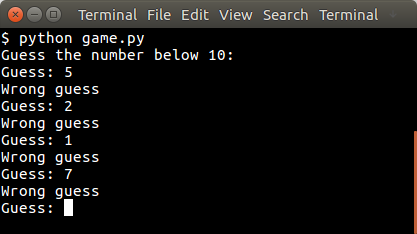# Simple text game with Python

In this article we will demonstrate how to create a simple guessing game.
The goal of the game is to guess the right number.

Example
An example run below:Simple text game with Python

You may like
Python Game Development for Beginners

## Random number

The user will be asked to guess the random number. We first pick the random number:

The randint() function will pick a pseudo random number between 1 and 10. Then we have to continue until the correct number is found:

## Python Guessing Game

The code below starts the game:

An example run:

## 4 thoughts on “Simple text game with Python”

1.- July 9, 2016

How do I play the game

1.- July 15, 2016

Execute it with Python. (python game.py), where game.py is one of the files above

2.- October 30, 2015

How do you print a random float?

1.- October 31, 2015

Hi Nathan,

You can get a random float using the random.uniform() function.
Example program generating a float between 1.1 and 5.5:

`import random x = random.uniform(1.1,5.5)print(x)`